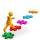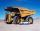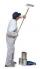Tank 9

The tank with volume V is filled with one pump for three hours and by second pump for 5 hours. When both pumps will run simultaneously calculate:

a) how much of the total volume of the tank is filled in one hour
b) for how long is the tank full

Result

a =  0.53
b =  1.875 h

Solution:

1*(1/3+1/5) = a
b(1/3+1/5)=1

15a = 8
8b = 15

a = 815 ≈ 0.533333
b = 158 = 1.875

Calculated by our linear equations calculator.

Leave us a comment of example and its solution (i.e. if it is still somewhat unclear...):Be the first to comment!To solve this example are needed these knowledge from mathematics:

Do you have a linear equation or system of equations and looking for its solution? Or do you have quadratic equation?

Next similar examples:

1. Belgium vs ItalyBelgium played a match with Italy and Belgium win by 2 goals. The match fell a total 6 goals. Determine the number of goals scored by Belgium and by Italy.
2. Rabbits 3Viju has 40 chickens and rabbits. If in all there are 90 legs. How many rabbits are there with Viju?
3. Hectoliters of waterThe pool has a total of 126 hectoliters of water. The first pump draws 2.1 liters of water per second. A second pump pumps 3.5 liters of water per second. How long will it take both pumps to drain four-fifths of the water at the same time?
4. Three peopleThree people start doing a work at a same time. The first worked only 2 hours. The second ended 3 hours before the end. On an individual basis, it would take the first time to do the work 10 hours, second 12 hours and 15 hours third. How many hours did it.
5. Journey to schoolOn the way to school I went steady step. In the first half of the journey, I counted every second step, in the second half every third. How many steps I do when I went to school if I counted double step are 250 more than three steps?
6. Father and daughterWhen I was 11 my father was 35 year old. Now, father has three times older then me. How old is she?
7. Father and sonWhen I was 11, my father was 35 years old. Today, my father has three times more years than me. How old is she?
8. Three figures - numbersThe sum of three numbers, if each is 10% larger than the previous one, is 662. Determine the figures.
9. SummerjobThree students participated in the summerjob. Altogether they earn 1780, -. Peter got a third less than John and Paul got 100,- more than Peter. How much got every one of them?
10. TrucksTruck take you the some amount of clay in 30 hours. Second truck in 40 hours. Trucks carted the clay away some time together then the second truck broke down and the first one completed the job in five hours. What time worked together?
11. Painters 4One painter will do the job for 12 days. After a 4 1/3 day he comes second to help him and complete work together for 8 days. How long would work the second painter himself?
12. 13 ticketsA short and long sightseeing tour is possible at the castle. Ticket for a short sightseeing circuit costs CZK 60, for a long touring circuit costs CZK 100. So far, 13 tickets have been sold for 1140 CZK. How much did you pay for tickets for a short tour?
13. Dining roomThe dining room has 11 tables (six and eight seats). In total there are 78 seats in the dining room. How many are six-and eight-seat tables?
14. Football seasonDalibor and Adam together scored 97 goals in the season. Adam scored 9 goals more than Dalibor. How many goals scored each?
15. The largerThe larger of two numbers is nine more than four times the smaller number. The sum of the two numbers is fifty-nine. Find the two numbers.
16. Equations - simpleSolve system of linear equations: x-2y=6 3x+2y=4
17. Six yearsIn six years Jan will be twice as old as he was six years ago. How old is he?